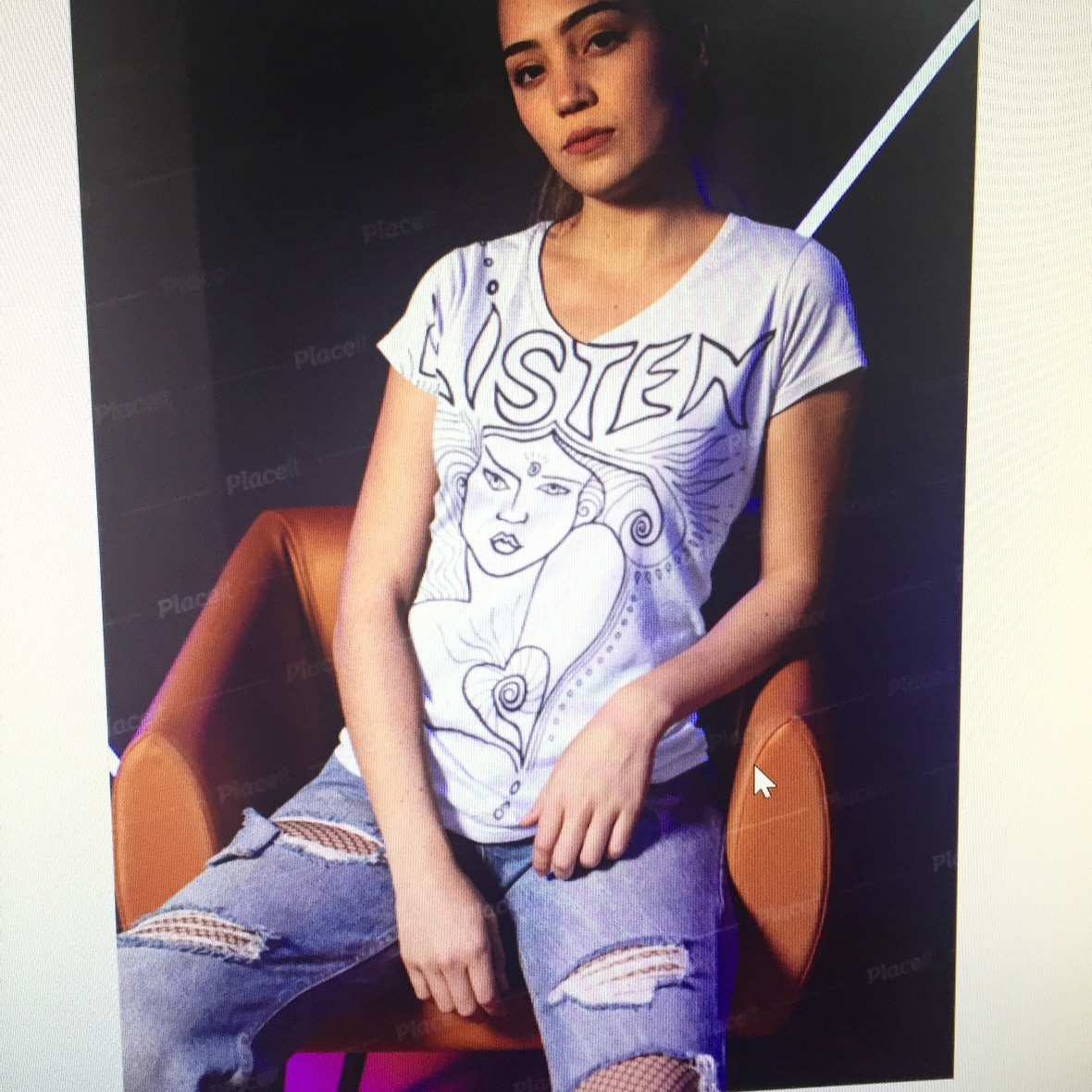Like   Tweet   Pin   +1   in## Sweet one,

I've got a quick favour to ask, and I really hope you will respond.

I'm exploring ideas for a clothing line, something I've not done in 30 years! (Never too old, right?)

Part of this vision includes artwork from my She Reflects Colouring Book. I'm going to start with 3 or 4 images to begin. They might be printed (silver or gold on dark fabric?), with beading, embroidery and/or studs. All options are on the table. (My muse hasn't given me full instructions yet!)

## Here's where you come in...

Since I love ALL my She Reflects drawings, and you are a super savvy consumer who knows exactly what you LOVE and what you would buy and wear, I need your precious feedback.

Please take a quick look below at 13 designs. Imagine each one on an article of clothing - let's say, a top, jacket or dress.

## Would YOU wear any of them?

If so, CLICK the image/s you LOVE. Choose up to 4 max.

Please make your selections by May 8.

Your clicks will be tallied up on the back-end, and I'll let you know which designs rose to the top. I can't wait to see what you pick.

(Feedback at erica@ericaross.com is also welcomed.)

Thanks for being a part of my process!

Erica

PS. If you don't click on any designs, I'll assume you WOULDN'T wear them, yes? So please take a moment to respond if you WOULD.

 table div table+table+table div table{width:100%;padding:0}table div table+table+table div table table{padding:0;float:left!important;width:54.205%!important}table div table+table+table div table td{padding-left:51px;padding-right:51px}table div table+table+table div table table td{padding-left:0;padding-right:20px}table div table+table+table div table table+table{float:left!important;width:45.795%!important}table div table+table+table div table table+table td{padding-left:0;padding-right:0}/* styles */
 table div table+table+table+table div table{width:100%;padding:0}table div table+table+table+table div table table{padding:0;float:left!important;width:52.494%!important}table div table+table+table+table div table td{padding-left:54px;padding-right:54px}table div table+table+table+table div table table td{padding-left:0;padding-right:20px}table div table+table+table+table div table table+table{float:left!important;width:47.506%!important}table div table+table+table+table div table table+table td{padding-left:0;padding-right:0}/* styles */
 table div table+table+table+table+table div table{width:100%;padding:0}table div table+table+table+table+table div table table{padding:0;float:left!important;width:53.186%!important}table div table+table+table+table+table div table td{padding-left:61px;padding-right:61px}table div table+table+table+table+table div table table td{padding-left:0;padding-right:20px}table div table+table+table+table+table div table table+table{float:left!important;width:46.814%!important}table div table+table+table+table+table div table table+table td{padding-left:0;padding-right:0}/* styles */
 table div table+table+table+table+table+table div table{width:100%;padding:0}table div table+table+table+table+table+table div table table{padding:0;float:left!important;width:52.369%!important}table div table+table+table+table+table+table div table td{padding-left:64px;padding-right:64px}table div table+table+table+table+table+table div table table td{padding-left:0;padding-right:20px}table div table+table+table+table+table+table div table table+table{float:left!important;width:47.631%!important}table div table+table+table+table+table+table div table table+table td{padding-left:0;padding-right:0}/* styles */
 table div table+table+table+table+table+table+table div table{width:100%;padding:0}table div table+table+table+table+table+table+table div table table{padding:0;float:left!important;width:54.145%!important}table div table+table+table+table+table+table+table div table td{padding-left:72px;padding-right:72px}table div table+table+table+table+table+table+table div table table td{padding-left:0;padding-right:20px}table div table+table+table+table+table+table+table div table table+table{float:left!important;width:45.855%!important}table div table+table+table+table+table+table+table div table table+table td{padding-left:0;padding-right:0}/* styles */
 table div table+table+table+table+table+table+table+table div table{width:100%;padding:0}table div table+table+table+table+table+table+table+table div table table{padding:0;float:left!important;width:48.958%!important}table div table+table+table+table+table+table+table+table div table td{padding-left:73px;padding-right:73px}table div table+table+table+table+table+table+table+table div table table td{padding-left:0;padding-right:20px}table div table+table+table+table+table+table+table+table div table table+table{float:left!important;width:51.042%!important}table div table+table+table+table+table+table+table+table div table table+table td{padding-left:0;padding-right:0}/* styles */
 table.module-8{width:39.62%;padding:0}table div table+table+table+table+table+table+table+table+table div table{width:39.62%;float:none;margin-left:auto;margin-right:auto;padding:0}table div table+table+table+table+table+table+table+table+table div table a{border:0 none;text-decoration:none}table div table+table+table+table+table+table+table+table+table div table img{width:100%!important;border:0 none;text-decoration:none}table div table+table+table+table+table+table+table+table+table div table td{width:100%;padding:0}/* styles */
 table div table+table+table+table+table+table+table+table+table+table div table{width:100%;padding:0}table div table+table+table+table+table+table+table+table+table+table div table img{width:96.23%;padding:0;float:none}table div table+table+table+table+table+table+table+table+table+table div table td{width:100%;padding:0 1.88% 18px}/* styles */table div table+table+table+table+table+table+table+table+table+table+table div table{width:100%;padding:0}table div table+table+table+table+table+table+table+table+table+table+table div table img{width:96.23%;padding:0;float:none}table div table+table+table+table+table+table+table+table+table+table+table div table td{width:100%;padding:0 1.88% 18px}/* styles *//* styles */ Follow me on Twitter, Instagram and Pinterest Follow me on Spotify Find me on Facebook Watch me on YouTube
 table div table+table+table+table+table+table+table+table+table+table+table+table+table+table+table+table+table div table{width:100%;padding:0}table div table+table+table+table+table+table+table+table+table+table+table+table+table+table+table+table+table div table img{width:96.23%;padding:0;float:none}table div table+table+table+table+table+table+table+table+table+table+table+table+table+table+table+table+table div table td{width:100%;padding:0 1.88% 18px}/* styles *//* styles */ If you found value and joy in this Love Letter, please SHARE it.